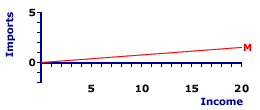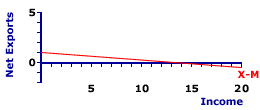Saturday  October 1, 2022
 AmosWEB means Economics with a Touch of Whimsy!BUSINESS TRANSFER PAYMENTS: A payment by the business sector to the household sector without any corresponding production or expectations of production. Business transfer payments are essentially gifts, or subsidies, made to the household sector from the business sector. This is one of several key differences between national income (the resource cost of production) and gross/net domestic product (the market value of production). For further discussion of this point, see gross domestic product and national income or net domestic product and national income. business transfer payments, abbreviated BTP, tend to be quite small, invariably less than 1% of gross domestic product.MARGINAL PROPENSITY TO IMPORT:

The change in imports purchased from the foreign induced by a change in income or production (national income or gross domestic product). The marginal propensity to import (abbreviated MPM) is another term for the slope of the imports line and is calculated as the change in imports divided by the change in income or production. The MPM plays a role in Keynesian economics. It augments the slope of the aggregate expenditures line and is part to the multiplier process. A related marginal measure is the marginal propensity to consume.
The marginal propensity to import (MPM) indicates the extent to which imports are induced by changes in income or production. If, for example, the MPM is 0.1, then each dollar of extra income in the economy induces 10 cents of imports.

The marginal propensity to import is important to the study of Keynesian economics. First, the MPM reflects induced imports. Second, the MPM is the slope of the imports line, which means it is the negative of the slope of the net exports line and makes it important to the slope of the aggregate expenditures line, as well. Third, the MPM affects the multiplier process and affects the magnitude of the expenditures and tax multipliers.

### Imports From Exports

Imports are important to the analysis of aggregate expenditures as part of net exports. Net exports are the difference between exports and imports, or exports minus imports. Exports are goods produced by the domestic economy and purchased by the foreign sector. Imports are goods produced by the foreign sector and purchased by the domestic economy (that is, the domestic household, business, and government sectors).

While exports are unrelated to domestic income or production, imports purchased from the foreign sector are induced by the level of domestic income and production. As household consumption is induced by income, so too are imports. When the household sector receives more income, it increases consumption expenditures, part of which are used to purchase imports. More income, means more consumption and more imports.

Of course, imports also consist of investment expenditures by the business sector and government purchases by the government sector. Both of these are induced by income and production, as well. And both of these are used to purchase imports.

Hence, imports are induced by domestic income and production. However, because imports are subtracted from exports to derive net exports, an induced increase in imports means an induced decrease in net exports.

### The MPM Formula

The standard formula for calculating marginal propensity to import (MPM) is:
 MPM = change in importschange in income
This formula has a couple of interpretations.
• First, it quantifies induced imports and induced net exports, that is, how many imports and net exports are induced by extra dollar. If domestic income or production changes by \$1, then imports change by the value of the MPM. Income induces the change in imports at a rate measured by the MPM. Moreover, because imports reduce net exports, net exports change at a rate equal to the NEGATIVE of the MPM.

• Second, the MPM is actually a measure of the slope of the imports line that plots the relation between imports and income. The measurement of slope is generally given as the "rise" over the "run." For the imports line, the rise is the change in imports and the run is the change in income. And once again, because imports reduce net exports, the slope of the net exports is equal to the NEGATIVE of the MPM.

### Slopes Of Two Lines

Imports LineNet Exports LineThe marginal propensity to import is another term for the slope of the imports line. It also shows up as the negative of the slope of the net exports line. This can be demonstrated and illustrated using the exhibit to the right. The top panel presents the imports line, labeled M, and the bottom panel contains the net exports line, labeled X-M. The net exports line is derived by subtracting the imports line from a constant amount of exports equal to \$2 trillion.

The imports line is positively sloped, indicating that greater levels of income generate greater imports from the foreign sector. The net exports line is negatively sloped, indicating that greater levels of income generate net exports.

This imports line in the top panel reflects a plot of the following imports equation:

 M = 0 + 0.075Y
where: M is imports and Y is income or production (national income or gross domestic product).

In particular, as specified in this imports equation, the slope of this imports line is equal to 0.075. This slope value indicates that each \$1 change in income induces a \$0.075 change in imports. In general, slope is calculated as the "rise" over the "run," that is, the change in the variable on the vertical axis (imports) divided by the change in the variable on the horizontal axis (production or income).

The change in imports divided by the change in income or production is the specification of the marginal propensity to import. That is, the slope of the imports line is the marginal propensity to import.

This net exports line in the bottom panel reflects a plot of the following net exports equation:

 NX = 1 - 0.075Y
where: NX is net exports and Y is again income or production.

The slope of this net exports line is equal to -0.075, which indicates that each \$1 change in income induces an opposite \$0.075 change in net exports. A \$1 increase in income generates a \$0.075 decrease in net exports. That is, the slope of the imports line is the negative of the marginal propensity to import.

To highlight the relation between MPM and the slopes of the imports and net exports lines, click the [Slope] button.

Moreover, because both lines are straight, their slopes are constant over the entire range of income, meaning the marginal propensity to import is also constant.

### Multiplier

The marginal propensity to import, like other expenditure marginals, affect the multiplier process. The multiplier measures the magnified change in aggregate production (gross domestic product) resulting from a change in an autonomous variable (such as investment). While the marginal propensity to consume is the most important marginal affecting the multiplier process, the marginal propensity to import also enters the picture.

The basic multiplier process results because a change in production (such as what occurs when autonomous investment expenditures purchase capital goods) generates income, which then induces consumption. However, the resulting consumption is also an expenditure on production, which generates more income, which induces more consumption. This next round of consumption also triggers a change in production, which generates even more income, and which induces even more consumption.

And on it goes, round after round. The end result is a magnified, multiplied change in aggregate production initially triggered by the change autonomous investment, but amplified by the change in induced consumption.

The multiplier process with induced consumption is augmented by induced imports. The change in production and income generated by the autonomous change in investment induces changes in both consumption AND IMPORTS. However, because imports reduce net exports, the increase in consumption expenditures on production are partially offset by the decrease in net exports. The resulting change production is less than what it would be without induce imports.

The MPM enters into the process along with the marginal propensity to consume (MPC) because it determines the degree to which induced imports, through induced net exports, diminish the induced change in consumption. If the MPM is greater, then the multiplier process is less.

This connection between the multiplier process, the marginal propensity to consume, and the marginal propensity to import is illustrated in the standard formula for an expenditures multiplier:

 expendituresmultiplier = 1(1 - (MPC - MPM))
An increase in the marginal propensity to import increases the value of the denominator on the right-hand side of the equation, which then decreases the overall value of the fraction and thus the size of the multiplier.

For example, given a marginal propensity to consume of 0.9, a marginal propensity to import of 0.1 results in a multiplier of 5. In contrast, a larger marginal propensity to import of 0.15 results in a smaller multiplier of 4.

### Other Marginals

The marginal propensity to import is one of several marginals that enters into the study of Keynesian economics. In fact, all induced variables have corresponding marginals that quantify the impact of income changes.

Here a few of the more important marginals:

• Marginal Propensity to Consume: The most important marginal in the study of Keynesian economics is the marginal propensity to consume (MPC). This embodies the fundamental psychological law indicating that an increase in income induces changes in consumption. The MPC is the slope of the consumption line and thus forms the foundation of the slope of the aggregate expenditures line. This marginal is also key to the magnitude of the multiplier process.

• Marginal Propensity to Save: The flip side of consumption is saving. The fundamental psychological law indicates that an increase in income induces changes in both consumption and saving. The marginal propensity to save (MPS) quantifies the saving part of this relation. It indicates the change in saving resulting from a change in income. In fact, if the MPC and MPS are calculated based on after-tax disposable income, then the two marginals sum to one: MPM + MPS = 1.

• Marginal Propensity to Invest: Consumption is not the only one of the aggregate expenditures induced by income and with a corresponding marginal. The marginal propensity to invest (MPI) is the change in investment induced by a change in income. The induced change in investment is not nearly as big as consumption, but it does affect the slope of the aggregate expenditures line and the size of the multiplier.

• Marginal Propensity for Government Purchases: Government purchases, like imports, is also induced by income and has a corresponding marginal. The marginal propensity for government purchases (MPG) is the change in government purchases induced by a change in income. The induced change in government purchases is related to the induced change in tax collections, and while it also small compared to imports, but it too affects the slope of the aggregate expenditures line and the size of the multiplier.

 <= MARGINAL PROPENSITY TO CONSUME MARGINAL PROPENSITY TO INVEST =>Recommended Citation:

MARGINAL PROPENSITY TO IMPORT, AmosWEB Encyclonomic WEB*pedia, http://www.AmosWEB.com, AmosWEB LLC, 2000-2022. [Accessed: October 1, 2022].

Check Out These Related Terms...

Or For A Little Background...

And For Further Study...
Search Again?GRAY SKITTERY[What's This?] Today, you are likely to spend a great deal of time at a crowded estate auction looking to buy either a country wreathe or galvanized steel storage shelves. Be on the lookout for fairy dust that tastes like salt.Your Complete ScopeA scripophilist is one who collects rare stock and bond certificates, usually from extinct companies."The truth is not for all men, but only for those who seek it. "-- Ayn Rand, writerSAFEXSouth African Futures ExchangeA PEDestrian's Guide Xtra CreditTell us what you think about AmosWEB. Like what you see? Have suggestions for improvements? Let us know. Click the User Feedback link.| | | | | | | | | | |
| | | |

Thanks for visiting AmosWEB